• Sometimes SVM considered by many as Large Margin Classifier
• What is it by mean? Given better understanding about SVM hypothesis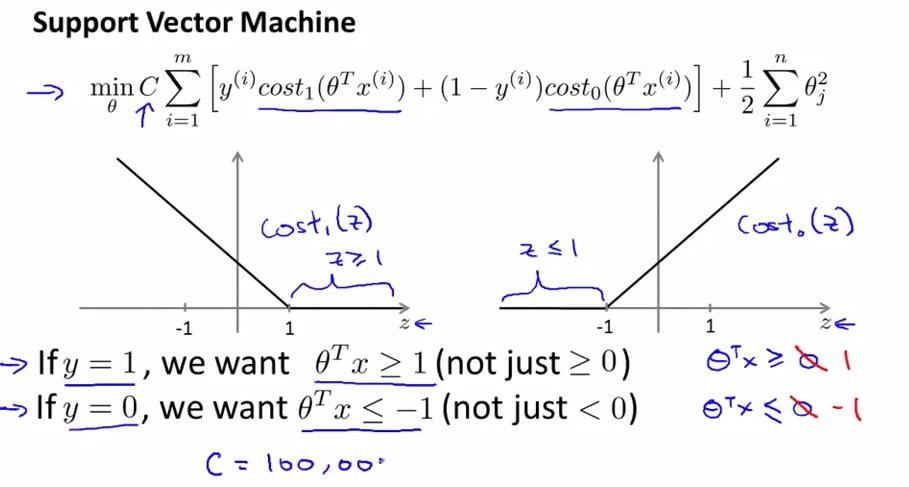• SVM then even extending the threshold in logistic regression so that SVM has even more safer threshold than logistic regression.
• By means if in logistic regression if set the hyphotesis to be equal or larger than zero, it just barely to get there. SVM wants to get stronger assurance of the predictions. So instead of 0, SVM with y = 1 wants to be >= 1, where y=0, hypothesis <= -1
• What is the consequences? What if C set to be much larger value?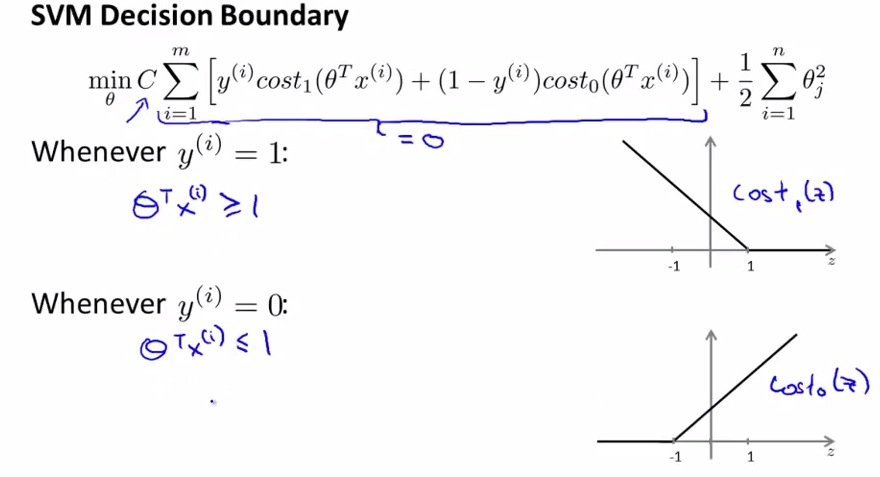• Intuitively we want cost function to be equal or less than zero. The goal of learning algorithm is minimizing the cost function.
• Since C acts the opposites from lambda, we want to minimize the cost function by making C very large value.
• Again, SVM optimization problem is the boundary as conditioned above.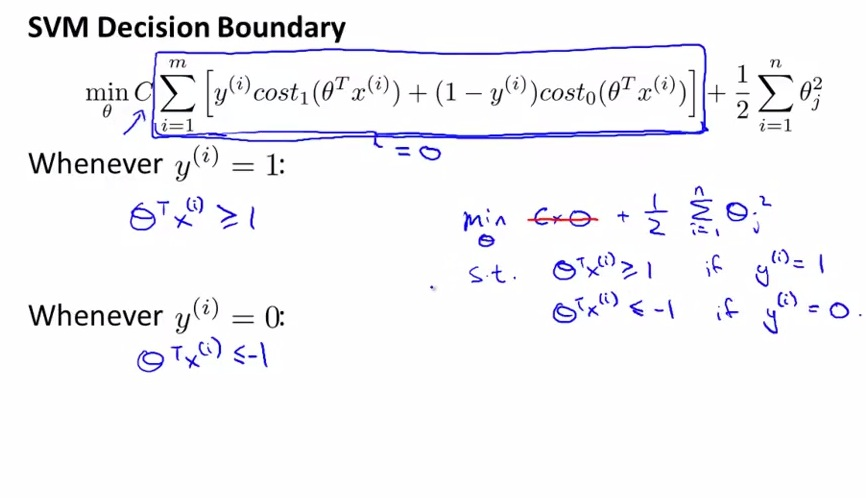• By minimizing the cost function until the CxA is equal to zero. we left with regularization term formula.
• And by minimizing theta, we want the final hypothesis is as stated above
• Next, how is decision boundary performed by SVM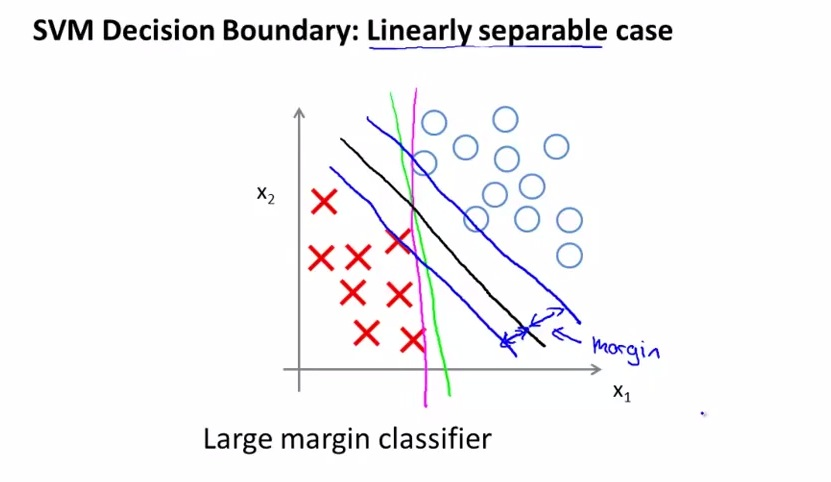• This is by means SVM often called Large margin classifier.
• Logistic regression may choose line drawn by green or magenta color. This is awfully close to the training examples and not perform a good solution for our learning algorithms.
• SVM seems like making a better job for decision boundary(mark by black color). It chooses line with the largest margin, make it more robust compared to logistic regression.The optimization problem provided by the earlier slides will lead to this margin, to be detailed in the next video.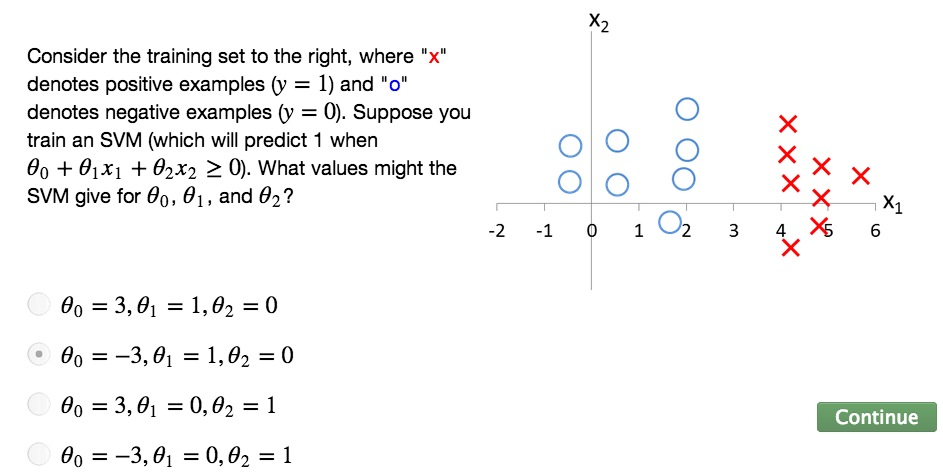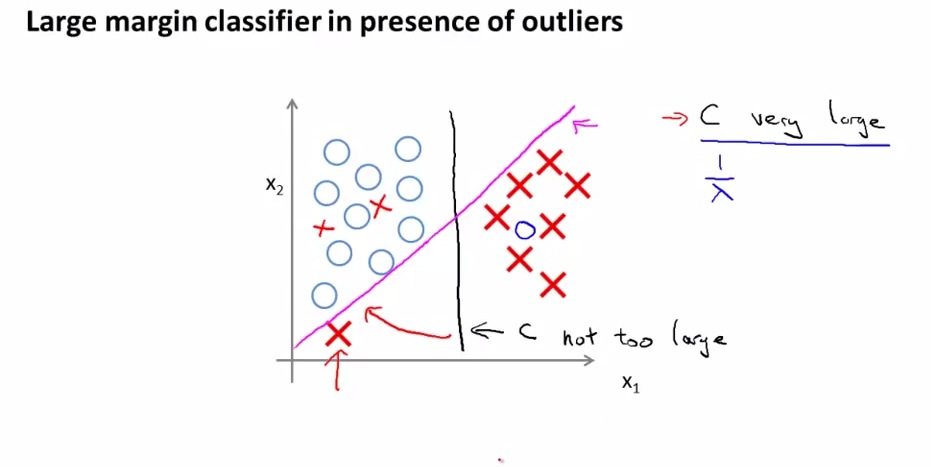• The second test case will show how C affects the function of our learning algorithms
• The C will be valued by some huge value, for example 100.000
• SVM is very sensitive to the outliers. Here SVM has manage an excellent job by drawn a black line classifier with large margin. But then there's an outliers drawn at the bottom left.
• Remember that C acts as contradiction of lambda. By making C very large, it will overfit the data. SVM will then change the line to magenta, making it no longer ideal classifier.
• What we want to is not making C very lage, will then making it ignoring outliers, as well as non-separable by linear outliers, as shown by outliers drawn among the blue circles.
• With ideal C value, SVM will retain the perfect classifier drawn by black line eventhough there's outliers

• Next discused more how the conditioned classifer will eventually lead to large margin classifier.